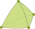#### Profile of Xuefeng LIU (xfliu)

Xuefeng LIU   @ Niigata University   HP: http://www.xfliu.org

My research focuses on numerical methods for partial differential equations.

####NS.SolutionVerification : Solution verification for the Navier-Stokes equation [Public]

By xfliu  ( )

Demonstration of the solution verification for the Naiver-Stokes equation over 3D domains.

####Hypercircle : The hypercircle method in the error estimation for FEM [Public]

By xfliu  ( )

This project describes how to obtain explicit a priori error estimation for FEM solution by using the hypercircle method.

####StokesEigProblem [Public]

By xfliu  ( )

The eigenvalue estimation for Stokes operator.

####vfem : Verified Finite Element Method Library [Public]

By xfliu  ( )

The Verified Finite Element Method (VFEM) library

####StokesEquation [Public]

By xfliu  ( )

The a priori error estimation for the FEM solution to Stokes equation over 3D domain.

####ProjectConstantCR [Public]

By xfliu  ( )

The projection error constant estimation for Crouzeix-Raviart FEM.

####OctaveInterval : Example of Interval package in Octave [Public]

By xfliu  ( )

The project show several examples about interval computing in Octave.

####EigenVecEstimation [Public]

By xfliu  ( )

This project shows a demo of the algorithm of the a posteriori error estimation for eigenvector of eigenvalue problems, such as, the matrix eigenvalue problem, the Laplacian eigenvalue problem.

####EigenvalueBound [Public]

By xfliu  ( )

The explicit lower bound and upper bound for the Laplace differential operator are obtained by using the conforming and non-conforming finite element method.